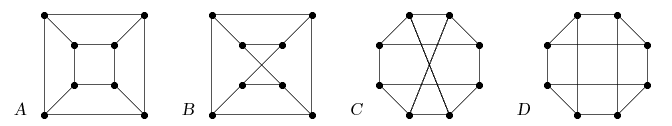MA010 Graph Theory (an online guide)

# Lecture 1: What is a graph

Outline• What is a graph
vertices (sometimes "nodes") and edges, simple graphs, cycles, paths, complete graphs
• Vertex degrees
vertex degree (also minimal and maximal), degree sequence of a graph (score) and its properties
• Subgraphs and isomorphism
subgraph and induced subgraph, cycles (triangles) and paths in a graph, cliques and independent sets;

isomorphism between two graphs, how to recognize (non-)isomorphic graphs
• Digraphs and Multigraphs
directed graphs (with arrows), multigraphs (with multiple edges and loops)

Goals

This is the basic introduction which shows what is a graph and what are the few basic terms needed to describe a graph (its vertices and edges, subgraphs, and isomorphism with other graphs). Students should learn about vertex degrees and the properties of a degree sequence (score) of a graph. Then about subgraphs and induced subgraphs in a graph (when, and how many times, our graph contains a specific subgraph, say a triangle or a path of length four...). The most important knowledge or ability from the first lecture (so important that it will be repeated also in the tutorials of some next lectures) is to recognize the relation of isomorphism of graphs (i.e. decide whethe given pair(s) of graphs are isomorphic or non-isomorphic), meaning that the presented graphs are "essentially the same".Graphs versus Multigraphs

It is not a big deal, but all students should understand from the beginning that there exist graphs which we mean simple (edges are two-element subsets, hence no bunches of parallel edges and no loops are possible), and then there are multigraphs in which a bunch of edges may share the same pair of endvertices (parallel edges) and even an edge with both ends in the same vertex may exist (called a loop). When we say a graph, we mean simple graph!

Pictures of graphs

When dealing with graphs (on a paper, not in a computer), the best way to "get grip" on it is to draw a nice graph picture. One of the early goals of our course is to teach you to draw graphs nicely, and see their properties in the pictures. Often, one has to try several pictures of the same graph before (s)he finds the right one - the one on which the answer or solution appears obvious. Likewise the next example (how many nonisomorphic graphs can you see?)...Lecture 1 slides EN
These are the MA010 lecturing slides provided for students in a printable format.
Slidy lekce 1 CZ
Výukové slidy MA010 v češtině. Doplňkové (přesto obsahující vše podstatné), neboť od 2009 probíhá výuka primárně v angličtině.Course book  [Invitation to Discrete Mathematics (by Jiří Matoušek, Jaroslav Nešetřil)]

Study Sections 4.1 (from page 109), starting part of 4.2, and whole 4.3.

Remarks
In the basic graph terminology, there exists one annoying ambiguity -- with the notation Pn for a path, some authors mean the index n to be the number of vertices, not the length. We stick with the course book and thus Pn is the path of length n.

Older editions of the book (both English and Czech) lack one of the starting chapters, and so the graph theory chapters start with number 3, not 4 as referred above. Intelligent students can surely subtract 1 from the chapter number in such a case... Furthermore, some of the book sections, mainly concerning Eulerian graphs and shortest paths, are arranged differently in the older or Czech editions. All our references are based on the 2008 English edition (see on google books).

For practical illustration of some of the graph theory terms from our curriculum, we provide their interactive demonstrations in some lectures. These demonstrations usually use java which may slow down your browser, and so they are not shown by default. Open the respective subsections when you want to access the demonstrations.